In :
using PyPlot
using LinearAlgebra


Suppose we want to solve a simple optimization problem over $\mathbb{R}^d$ with objective function $$f(x) = \frac{1}{2} \| x \|^2.$$ Here, the gradient is $$f'(x) = x.$$

In :
function grad_f(x)
return x
end

Out:
grad_f (generic function with 1 method)

Suppose that our stochastic objective samples are of the form $$\tilde f(x) = \frac{1}{2} \| x \|^2 + x^T z$$ where $z \sim N(0,\sqrt{d} \cdot I)$ is a standard Gaussian random variable with expected norm-squared $1$. Then our gradient samples will be of the form $$\nabla \tilde f(x) = x + z.$$

In :
function sample_grad_f(x)
return x + randn(length(x)) / sqrt(length(x))
end

Out:
sample_grad_f (generic function with 1 method)
In :
function gradient_descent(x0, alpha, num_iters)
dist_to_optimum = zeros(num_iters)
x = x0
for t = 1:num_iters
x = x - alpha * grad_f(x)
dist_to_optimum[t] = norm(x)
end
return dist_to_optimum
end

dist_to_optimum = zeros(num_iters)
x = x0
for t = 1:num_iters
x = x - alpha * sample_grad_f(x)
dist_to_optimum[t] = norm(x)
end
return dist_to_optimum
end

Out:
stochastic_gradient_descent (generic function with 1 method)
In :
x0 = [5.0];
alpha = 0.1;
num_iters = 1000;

In :
plot(1:num_iters, gd_dist, 1:num_iters, sgd_dist);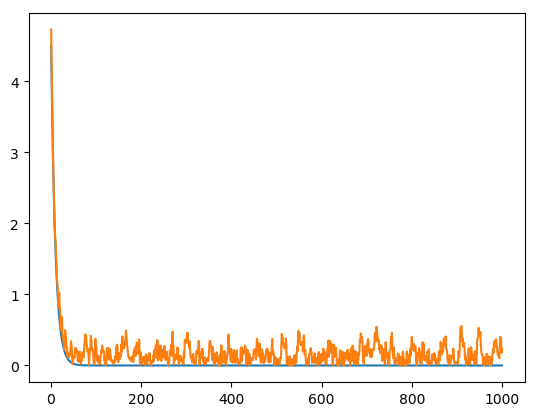In :
semilogy(1:num_iters, gd_dist, 1:num_iters, sgd_dist);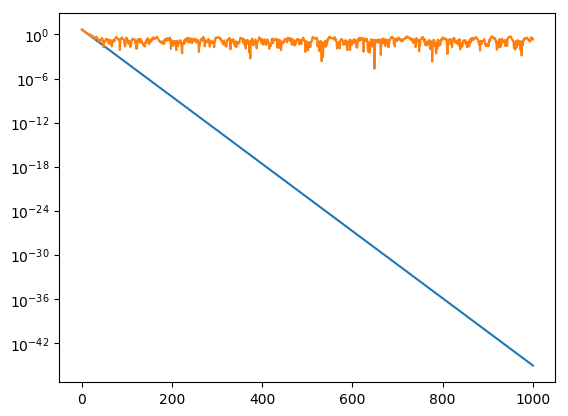In :
x0 = 2 * randn(100);
alpha = 0.1;
num_iters = 5000;

In :
plot(1:num_iters, gd_dist, 1:num_iters, sgd_dist);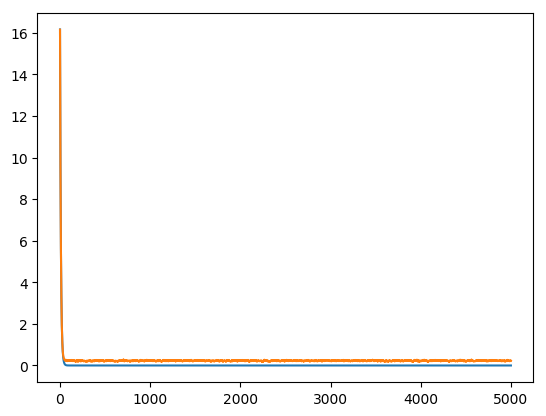In :
plot(1:num_iters, gd_dist, 1:num_iters, sgd_dist);
ylim([-0.1,0.6]);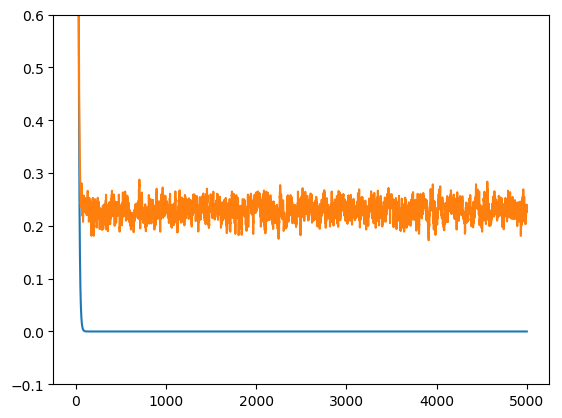In :
semilogy(1:num_iters, gd_dist, 1:num_iters, sgd_dist);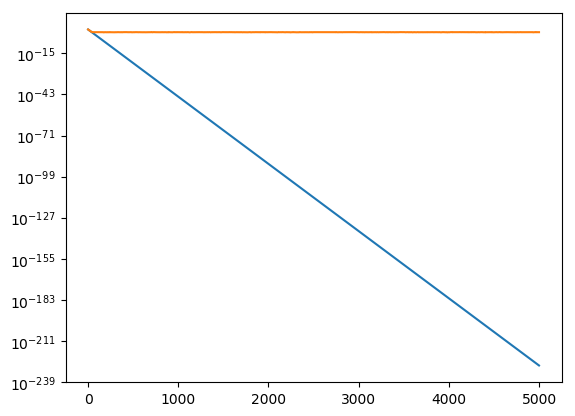In [ ]: